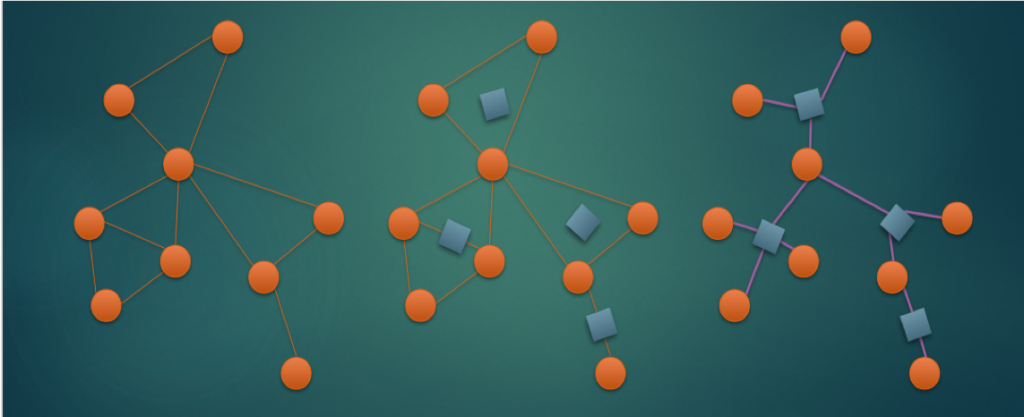## 圆方树（第一个是原图，第三个是原图对应的圆方树）

## 「BZOJ3331」「BJOI2013」压力

$1\leq n \leq 10^5，1 \leq m,q \leq 2*10^5$

#include
#include
#include
#include
#include
#include
#include
#include
#include
#include
#include
#include
#include

#define Re register
#define LL long long
#define U unsigned
#define FOR(i,a,b) for(Re int i = a;i <= b;++i)
#define ROF(i,a,b) for(Re int i = a;i >= b;--i)
#define SFOR(i,a,b,c) for(Re int i = a;i <= b;i+=c)
#define SROF(i,a,b,c) for(Re int i = a;i >= b;i-=c)
#define CLR(i,a) memset(i,a,sizeof(i))
#define BR printf("--------------------\n")
#define DEBUG(x) std::cerr << #x << '=' << x << std::endl

const int MAXN = 250000+5;
const int MAXM = 450000+5;

struct Edge{
int to,next;
}e1[MAXM<<1],e2[MAXM<<1];
float log2N;
int dfn[MAXN],low[MAXN],bel[MAXN],st[MAXN],top;
int f[MAXN],val[MAXN],tot,d[MAXN];
int N,M,Q;

}

}

void dfs1(int v){
static int ts = 0;
dfn[v] = low[v] = ++ts;st[++top] = v;
for(int i = head1[v];i;i = e1[i].next){
if(!dfn[e1[i].to]){
dfs1(e1[i].to);low[v] = std::min(low[v],low[e1[i].to]);
if(low[e1[i].to] >= dfn[v]){
tot++;int t = -1;
do{
if(d[v] <= (1<